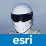# QueryPoint from end of the line....not start

133
2
06-26-2020 07:02 AMRegular Contributor

Is there a simple way to use GeometryEngine.Instance.QueryPoint to get a point a specified distance from the end of the line?  It seems to default to the start of the line??

Or do I just need to add some math logic to my code??

1 Solution

Accepted SolutionsbyEsri Regular Contributor

Hey Brian,

Yes you can simply reverse the orientation of your line geometry and do the querypoint using the original distance.

``````      var reverseGeom = GeometryEngine.Instance.ReverseOrientation(geometry as Multipart);
var qPoint = GeometryEngine.Instance.QueryPoint(reverseGeom, SegmentExtension.NoExtension, dac, AsRatioOrLength.AsLength);‍‍‍‍‍‍``````

or alternatively get the length of the geometry and work out the new query point distance.

``````      var length2D = GeometryEngine.Instance.Length(geometry);
var newDistance = length2D - originalDistance;
var qPoint = GeometryEngine.Instance.QueryPoint(geometry, SegmentExtension.NoExtension, newDistance, AsRatioOrLength.AsLength);‍‍‍‍‍‍‍‍‍``````
2 RepliesbyEsri Regular Contributor

Hey Brian,

Yes you can simply reverse the orientation of your line geometry and do the querypoint using the original distance.

``````      var reverseGeom = GeometryEngine.Instance.ReverseOrientation(geometry as Multipart);
var qPoint = GeometryEngine.Instance.QueryPoint(reverseGeom, SegmentExtension.NoExtension, dac, AsRatioOrLength.AsLength);‍‍‍‍‍‍``````

or alternatively get the length of the geometry and work out the new query point distance.

``````      var length2D = GeometryEngine.Instance.Length(geometry);
var newDistance = length2D - originalDistance;
var qPoint = GeometryEngine.Instance.QueryPoint(geometry, SegmentExtension.NoExtension, newDistance, AsRatioOrLength.AsLength);‍‍‍‍‍‍‍‍‍``````Regular Contributor

Hi Sean Jones‌.  I ended up just doing math on it, but it's good to know about the ReverseOrientation option.

Thanks!